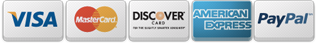# Queen Size Crosshatch-L02829*

Note: pattern is 18 x 110 inches.  For systems with p2p or line pattern capabilities:  Place 2 rows of crosshatch on screen, separate by one half the vertical height of 1 crosshatch diamond.  Stitch the two repeats, go back and fill in the space with p2p line.

To figure out the space:  count the total number of half diamonds vertically ( queen size crosshatch has 12.5 full diamonds, so multiply by 2 = 25). Take the total height of the pattern (default is 18 inches) divide by total number of half diamonds (25 for the queen size crosshatch).  The resulting answer is the amount of space needed between rows of the crosshatch (.72 for the queen size crosshatch).

This will work for any crosshatch pattern that has a full dimond on one side and a half diamond (vertically) on the other: total height of pattern divided by total number of half diamonds = space between rows to be able to fill the space using p2p line.

## \$20.00